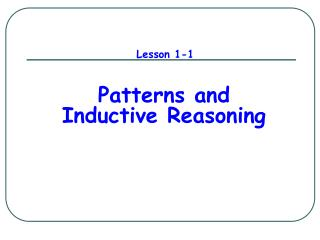DownloadDownload PresentationLesson 1-1

# Lesson 1-1

Télécharger la présentation## Lesson 1-1

- - - - - - - - - - - - - - - - - - - - - - - - - - - E N D - - - - - - - - - - - - - - - - - - - - - - - - - - -
##### Presentation Transcript

1. Lesson 1-1 Patterns and Inductive Reasoning

2. Inductive Reasoning Making conclusions/predictions based on patterns and examples. • Find the next two terms:3, 9, 27, 81, . . . • Draw the next picture: • Find the next two terms:384, 192, 96, 48, . . . 243, 729 24, 12

3. Making a Conjecture Make a conclusion based on inductive reasoning. Use the table to make a conjecture about the sum of the first six positive even numbers. 2 = 2 = 1·2 2 + 4 = 6 = 2·3 2 + 4 + 6 = 12 = 3·4 2 + 4 + 6 + 8 = 20 = 4·5 2 + 4 + 6 + 8 + 10 = 30 = 5·6 = 6·7 = 42

4. counterexample Counterexample (like a contradiction) An example for which the conjecture is incorrect. Conjecture: the product of two positive numbers is greater than either number.

5. Fun Patterns Find the next character in the sequenceJ, F, M, A, . . . January, February, March, April, May Find the next character in the sequenceS, M, T, W, . . . Sunday, Monday, Tuesday, Wednesday, Thursday Find the next character in the sequenceZ, O, T, T, F, F, S, S, . . . Zero, One, Two, Three, Four, Five, Six, Seven, Eight Find the next character in the sequence3, 3, 5, 4, 4, . . . One has 3 letters, Two has 3, Three has 5, Four has 4, Five has 5, Six has 3

6. Lesson 1-2 Points, Lines, and Planes

7. Point • A point does not have an actual size; it represents a location. • How to Sketch: Use dots • How to label: Use CAPITAL letters • Never name two points with the same letter (in the same sketch). A B A C

8. A B C Line • A set of points that extends infinitely in opposite directions and has no thickness or width. • How to sketch : use arrows at both ends. • How to name: 2 ways (1) small italics letter — line m(2) any two points on the line — • Never name a line using three points — m

9. Collinear Points Collinear points are points that lie on the same line. (The line does not have to be visible.) E D A B C F Collinear Non collinear

10. Planes • A plane is a flat surface that extends indefinitely in all directions. • How to sketch: Use a parallelogram (four sided figure) • How to name: 2 ways (1) Capital italics letter — Plane M (2) Any 3 noncollinear points in the plane — Plane: ABC/ ACB / BAC / BCA / CAB / CBA A M B C Horizontal Plane Vertical Plane Other

11. Different planes in a figure: A B Plane ABCD Plane EFGH Plane BCGF Plane ADHE Plane ABFE Plane CDHG Etc. D C E F H G

12. Coplanar Objects Coplanar objects (points, lines, etc.) are objects that lie on the same plane. The plane does not have to be visible. Are the following points coplanar? A, B, C ? Yes A, B, C, F ? No H, G, F, E ? Yes E, H, C, B ? Yes A, G, F ? Yes C, B, F, H ? No

13. Postulate An accepted statement or fact. You accept a postulate as true without proof; you try to determine if a conjecture is true or false.

14. t B A Postulate 1-1 Through any two points there is exactly one line.

15. B P A C D Postulate 1-2 If two lines intersect, then they intersect in exactly one point.

16. Postulate 1-3 If two planes intersect, then they intersect in exactly one line. B P A Plane P and Plane R intersect at the line R

17. Postulate 1-4 Through any three noncollinear points there is exactly one plane. Plane AFGD Plane ACGE Plane ACH Plane AGF Plane BDG Etc.

18. 3 Possibilities of Intersection of a Line and a Plane (1) Line passes through plane — intersection is a point. (2) Line lies on the plane — intersection is a line. (3) Line is parallel to the plane — no common points.